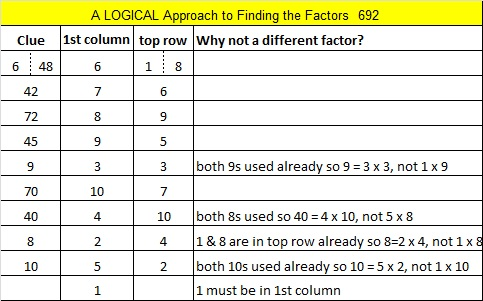# 1555 Two Turkeys Too Tough To Try?

### Today’s Puzzle:

Two turkeys too tough to try? That’s a six-word title made with alliteration and three homophones! It also describes the mystery-level turkey puzzles below. Those turkeys might look like identical twins at first glance, but if you look closely, you will see they are not quite the same.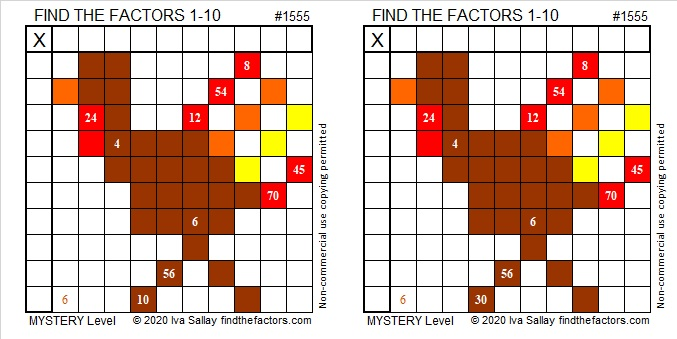Here are some questions to help you find a logical way to start either puzzle: Which two clues MUST use the two 6’s as factors? Are there any other clues that are multiples of 6? If so, what factors would those clues use?

### Factors of 1555:

• 1555 is a composite number.
• Prime factorization: 1555 = 5 × 311.
• 1555 has no exponents greater than 1 in its prime factorization, so √1555 cannot be simplified.
• The exponents in the prime factorization are 1 and 1. Adding one to each exponent and multiplying we get (1 + 1)(1 + 1) = 2 × 2 = 4. Therefore 1555 has exactly 4 factors.
• The factors of 1555 are outlined with their factor pair partners in the graphic below.### Another Fact about the Number 1555:

1555 is the hypotenuse of a Pythagorean triple:
933-1244-1555, which is (3-4-5) times 311.

# 1299 Is This Puzzle a Real Turkey?

Happy Thanksgiving, everyone!

Turkeys run but they cannot hide. They all will eventually end up on somebodies’ table. There doesn’t seem to be much of a mystery about that, but I’ve created a mystery level puzzle for today anyway. I promise it can be solved using logic and the basic facts in a 12 × 12 multiplication table.Print the puzzles or type the solution in this excel file: 12 factors 1289-1299

Now I’ll share some facts about the number 1299:

• 1299 is a composite number.
• Prime factorization: 1299 = 3 × 433
• The exponents in the prime factorization are 1 and 1. Adding one to each and multiplying we get (1 + 1)(1 + 1) = 2 × 2 = 4. Therefore 1299 has exactly 4 factors.
• Factors of 1299: 1, 3, 433, 1299
• Factor pairs: 1299 = 1 × 1299 or 3 × 433
• 1299 has no square factors that allow its square root to be simplified. √1299 ≈ 36.04164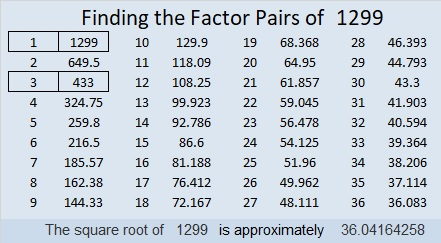1299 is the hypotenuse of a Pythagorean triple:
435-1224-1299 which is 3 times (145-408-433)

OEIS.org informs us that 8¹²⁹⁹ ≈ 1299 × 10¹¹⁷⁰. You can see it for yourself on a computer calculator!

# 965 Run, Turkey, Run!

Happy Thanksgiving, everyone!

I didn’t mean to make any Thanksgiving puzzles but after I created the puzzles this week, I could see some Thanksgiving-related pictures in the designs I had already made. This one is my favorite.

Run, Turkey, Run! For millions of turkeys today, it’s already too late.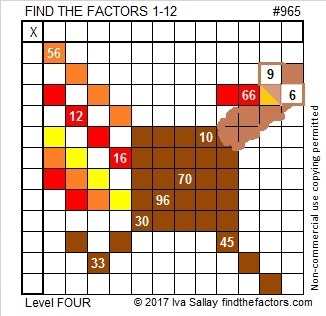Print the puzzles or type the solution in this excel file: 12 factors 959-967

Here is a little about the number 965:

965 is the sum of two squares two different ways:
26² + 17² = 965
31² + 2² = 965

So it is also the hypotenuse of FOUR Pythagorean triples, two of them primitives:
124-957-965, calculated from 2(31)(2), 31² – 2², 31² + 2²
387-884-965, calculated from 26² – 17², 2(26)(17), 26² + 17²
475-840-965 which is 5 times (95-168-193)
579-772-965 which is (3-4-5) times 193

• 965 is a composite number.
• Prime factorization: 965 = 5 × 193
• The exponents in the prime factorization are 1 and 1. Adding one to each and multiplying we get (1 + 1)(1 + 1) = 2 × 2 = 4. Therefore 965 has exactly 4 factors.
• Factors of 965: 1, 5, 193, 965
• Factor pairs: 965 = 1 × 965 or 5 × 193
• 965 has no square factors that allow its square root to be simplified. √965 ≈ 31.064449# 692 Happy Thanksgiving!

• 692 is a composite number.
• Prime factorization: 692 = 2 x 2 x 173, which can be written 692 = (2^2) x 173
• The exponents in the prime factorization are 2 and 1. Adding one to each and multiplying we get (2 + 1)(1 + 1) = 3 x 2  = 6. Therefore 692 has exactly 6 factors.
• Factors of 692: 1, 2, 4, 173, 346, 692
• Factor pairs: 692 = 1 x 692, 2 x 346, or 4 x 173
• Taking the factor pair with the largest square number factor, we get √692 = (√4)(√173) = 2√173 ≈ 26.30589.Today is Thanksgiving in the United States. Regardless of where we live, there are reasons to be grateful today and every other day. Corrie ten Boom was grateful even when her circumstances were horrible. Here’s a Thanksgiving-themed puzzle to solve:Sometimes color in a puzzle is a distraction. Here’s the same puzzle minus the color: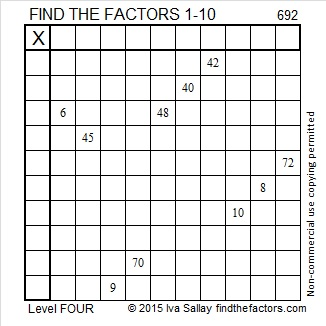Print the puzzles or type the solution on this excel file: 10 Factors 2015-11-23

————————————————————————————

Here’s a little more about the number 692:

Because 173 is one of its factors, 692 is the hypotenuse of Pythagorean triple 208-660-692. What is the greatest common factor of those three numbers?

692 is a palindrome in 2 bases:

• 221122 BASE 3; note that 2(243) + 2(81) + 1(27) + 1(9) + 2(3) + 2(1) = 692
• 848 BASE 9; note that 8(81) + 4(9) + 8(1) = 692

————————————————————————————